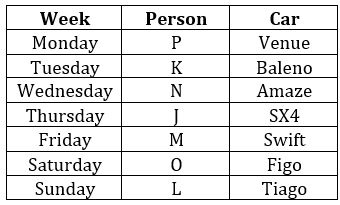Latest Banking jobs   »

# Reasoning Ability Quiz For FCI Phase I 2022- 30th December

Directions (1-5): Study the information carefully and answer the questions given below.
Seven persons are going for trip on seven different days of the week (Monday to Sunday). They all have different cars i.e., SX4, Venue, Figo, Amaze, Baleno, Swift and Tiago. All information is not necessarily in same order.
The person who has Swift go immediately before O. More than three persons go between O and P who has venue. P does not go on Tuesday. Two persons go between the one who has Swift and the one who has baleno. Equal number of persons goes before and after J who has SX4. As many persons go between K and J as many between J and O. N who has Amaze goes before L who has Tiago. M does not have figo.

Q1. Who among the following person goes on Friday?
(a) M
(b) O
(c) J
(d) L
(e) None of these

Q2. Who among the following person goes immediately before J?
(a) P
(b) K
(c) M
(d) O
(e) None of these

Q3. Who among the following person goes on Sunday?
(a) The one who has Figo
(b) The one who has Sx4
(c) The one who has Swift
(d) The one who has Tiago
(e) None of these

Q4. Four of the following five are alike in certain way based from a group, find the one that does not belong to that group?
(a) P-Amaze
(b) K-SX4
(c) N-Swift
(d) M-Tiago
(e) N-Figo

Q5. Who among the following person has Figo?
(a) P
(b) N
(c) J
(d) O
(e) K

Direction (6-10): Study the following alphanumeric series carefully and answer the questions given below:
S * 7 B \$ * G 2 8 U 1 & A V # F 3 5 H @ 5 Y 5 M 3 2 D 8 % N 2 4

Q6. How many such numbers are there which is immediately preceded by alphabets and immediately followed by symbols?
(a) One
(b) Two
(c) Three
(d) Four
(e) None

Q7. Which of the following element is 19th from right end of the series?
(a) #
(b) F
(c) 3
(d) V
(e) None of these

Q8. How many such symbols are there which is immediately preceded by numbers and immediately followed by vowels?
(a) Two
(b) None
(c) One
(d) Four
(e) Three

Q9. How many such alphabets are there which is immediately preceded and followed by same number?
(a) Three
(b) Two
(c) One
(d) Four
(e) None

Q10. If all numbers are eliminated from the series, then, which of the following element is 11th from right end of the series?
(a) V
(b) A
(c) &
(d) #
(e) None of these

Directions (11-15): Following questions are based on the five three-digit numbers given below.

758 856 918 824 594

Q11. If all the digits in each of the numbers are arranged in descending order within the number, then, which of the following number will become the lowest in the new arrangement of numbers?
(a) 758
(b) 856
(c) 918
(d) 824
(e) 594

Q12. If all the numbers are arranged in ascending order from left to right then, which of the following will be the sum of all the three digits of the number which is 3rd from the left in the new arrangement?
(a) 19
(b) 14
(c) 18
(d) 20
(e) None of these

Q13. What will be the resultant when second digit of the lowest number is multiplied with the second digit of the second highest number?
(a) 9
(b) 18
(c) 45
(d) 48
(e) None of these

Q14. If the positions of the second and the third digits of each of the numbers are interchanged then, how many odd numbers will be formed?
(a) None
(b) One
(c) Two
(d) Three
(e) Four

Q15. If one is added to the third digit of each of the numbers and one is subtracted to the second digit of each of the number then how many numbers thus formed will be divisible by three?
(a) None
(b) One
(c) Two
(d) Three
(e) Four

Solutions

Solution (1-5):
Sol.S1. Ans. (a)
S2. Ans. (e)
S3. Ans. (d)
S4. Ans. (e)
S5. Ans. (d)

S6. Ans. (b)
Sol. U1&, D8%
S7. Ans. (d)
S8. Ans. (c)
Sol. 1&A
S9. Ans. (c)
Sol. 5 Y 5
S10. Ans. (b)

S11. Ans. (d)
S12. Ans. (b)
S13. Ans. (c)
S14. Ans. (e)
S15. Ans. (c)#### Congratulations!Union Budget 2023-24: Free PDF